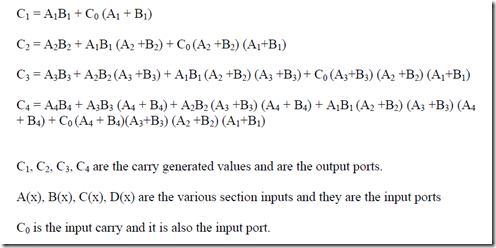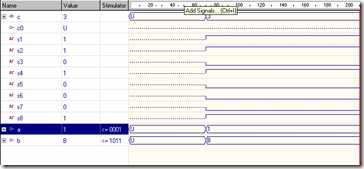Simulation of Look Ahead Carry Generator

Requirements:

• WARP
• Active HDL

Design:Program for the Look Ahead Carry Generator
library IEEE;
use IEEE.std_logic_1164.all;
port (
a: in STD_LOGIC_VECTOR (4 downto 1);
b: in STD_LOGIC_VECTOR (4 downto 1);
c0: in STD_LOGIC;
c: out STD_LOGIC_VECTOR (4 downto 1)
);
signal s1,s2,s3,s4,s5,s6,s7,s8:std_logic;
begin
s1 <= a(1) and b(1);
s2 <= a(1) or b(1);
s3 <= a(2) and b(2);
s4 <= a(2) or b(2);
s5 <= a(3) and b(3);
s6 <= a(3) or b(3);
s7 <= a(4) and b(4);
s8 <= a(4) or b(4);
c(1) <= s1 or (c0 and s2);
c(2) <= s3 or (s1 and s4) or (c0 and s2 and s4);
c(3) <= s5 or (s3 and s6) or (s1 and s4 and s6) or (c0 and s2 and s4 and s6);
c(4) <= s7 or (s5 and s8) or (s3 and s6 and s8) or (s1 and s4 and s6 and s8)
or (c0 and s2 and s4 and s6 and s8);